Cracking codes / The Merseburg drawing

# The Merseburg drawing

 Looking closer at the Merseburg drawing we will find te same consistencies. If we count the weights in the pendulum weights in the picture, we will find 5 square weights and 3 circular pendulum weights. Again we have 5 and 3 (totally 8).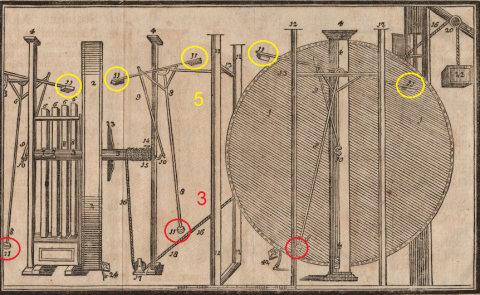When we look closer again, we see that all these weights have the value of 11.   Value of square weights :                  11 x 5 = 55 Value of the circular weights:             11 x 3 = 33 Value of all eight pendulum weights:   11 x 8 = 88   And the value of ORFFYRE = O+R+F+F+Y+R+E' = 88    The most important of all is that we have found the signs of a very elaborate code in the writings of Orffyreus, and we get a confirmation that we have understood their meaning properly.   Now, let us see how this discovered 5/3 appears as geometry in the same drawing. Remember that he even called himself ORFFYREUS 5/3   Looking closer at the drawing and the wheel itself, we can see that he has drawn tiny lines that has about a 30 degrees angle.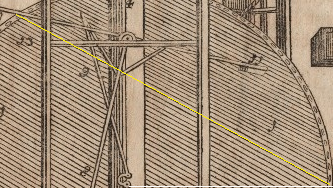If we complete this geometry, we get a triange: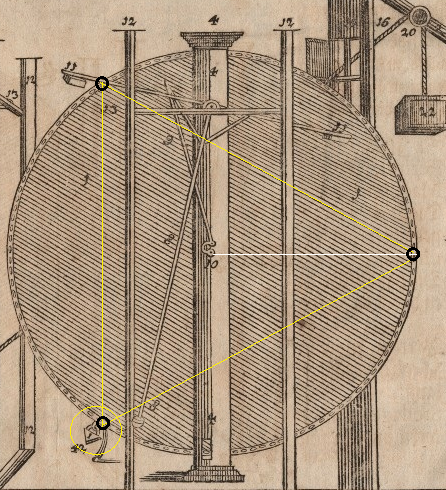The triangle meets the triangle shaped padlock. Now we can understand why he placed this padlock just here.   So if 3 represents a triangle, what about 5 then?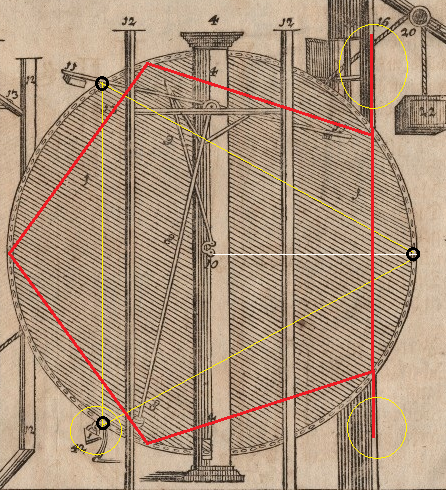We now see that the right wall, is placed in line with a pentagon, and we can see that this setting is just a horizontal version of the earlier found solutions.   So where is this proven code leading us?   When I get to publish my books you will find out.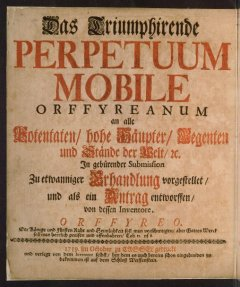Das Triumphierende
PERPETUUM MOBILE
ORFFYREANUM
1719